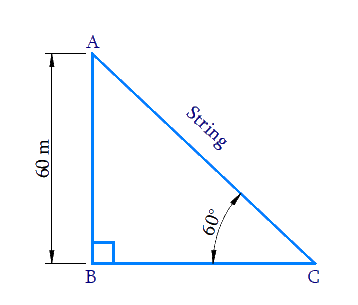# Ex.9.1 Q5 Some Applications of Trigonometry Solution - NCERT Maths Class 10

Go back to  'Ex.9.1'

## Question

A kite is flying at a height of $$60\,\rm{m}$$ above the ground. The string attached to the kite is temporarily tied to a point on the ground. The inclination of the string with the ground is $$60^\circ$$. Find the length of the string, assuming that there is no slack in the string.

Video Solution
Some Applications Of Trigonometry
Ex 9.1 | Question 5

## Text Solution

What is Known?

(i) Kite flying at a height $$= 60\,{\rm { m}}$$

(ii) Angle made by the string to the ground $$=60^\circ$$

What is Unknown?

Length of the stringReasoning:

Let the height of the flying kite as $$AB$$, length of the string as $$AC$$ and the inclination of the string with the ground as $$\angle C$$

Trigonometric ratio involving $$AB, AC$$ and $$\angle C$$  is $$\sin \theta$$

Steps:

In $$\Delta ABC$$,

\begin{align}\sin \,C &= \frac{{AB}}{{AC}}\\\sin \,{60^0} &= \frac{{60}}{{AC}}\\\frac{{\sqrt 3 }}{2} &= \frac{{60}}{{AC}}\\AC &= \frac{{60 \times 2}}{{\sqrt 3 }}\\&= \frac{{120}}{{\sqrt 3 }} \times \frac{{\sqrt 3 }}{{\sqrt 3 }}\\&= \frac{{120\sqrt 3 }}{3}\\&= {\rm{40}}\sqrt {\rm{3}} \,\end{align}

Length of  the string $$AC =$$ $$40 \sqrt{3} \mathrm{m}$$

Learn from the best math teachers and top your exams

• Live one on one classroom and doubt clearing
• Practice worksheets in and after class for conceptual clarity
• Personalized curriculum to keep up with school